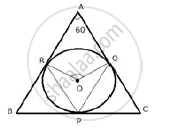Share

In a Triangle Abc, the Incircle (Centre O) Touches Bc, Ca and Ab at Points P, Q and R Respectively. Calculate: I)∠Qpr . - ICSE Class 10 - Mathematics

ConceptTangent Properties - If a Line Touches a Circle and from the Point of Contact, a Chord is Drawn, the Angles Between the Tangent and the Chord Are Respectively Equal to the Angles in the Corresponding Alternate Segments

Question

In a triangle ABC, the incircle (centre O) touches BC, CA and AB at points P, Q and R respectively. Calculate:

i)∠QPR .

SolutionThe incircle touches the sides of the triangle ABC and
OP ⊥ BC,OQ ⊥ AC,OR ⊥ AB

i) Now arc RQ subtends∠QOR at the centre and ∠QPR at the remaining part of the circle.

∴ ∠QPR = 1/2 ∠ QOR

⇒ ∠QPR = 1/2 xx120°

⇒ ∠ QPR = 60°

Is there an error in this question or solution?

Video TutorialsVIEW ALL 

Solution In a Triangle Abc, the Incircle (Centre O) Touches Bc, Ca and Ab at Points P, Q and R Respectively. Calculate: I)∠Qpr . Concept: Tangent Properties - If a Line Touches a Circle and from the Point of Contact, a Chord is Drawn, the Angles Between the Tangent and the Chord Are Respectively Equal to the Angles in the Corresponding Alternate Segments.
S